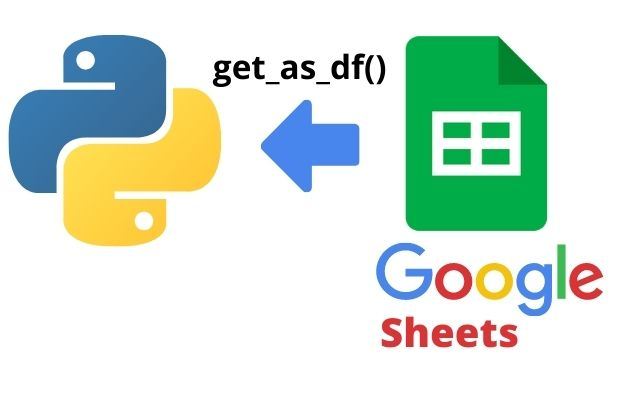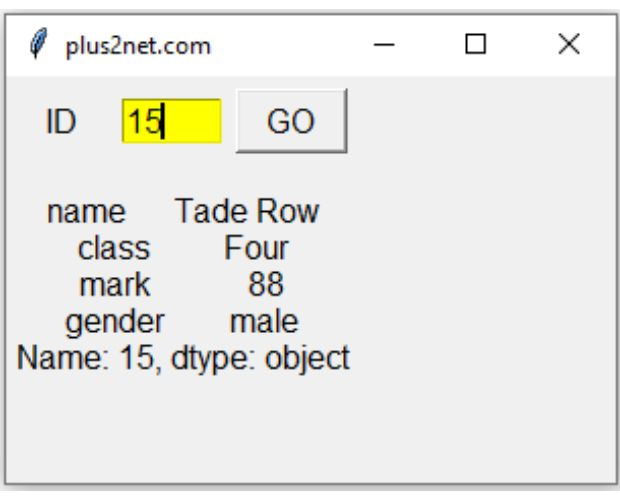Read Excel file and create DataFrame ( df ).
``````import pandas as pd
print(df.head()) # top five rows of the DataFrame``````
Output is here
``````   id        name  class  mark  gender
0   1    John Deo   Four    75  female
1   2    Max Ruin  Three    85    male
2   3      Arnold  Three    55    male
3   4  Krish Star   Four    60  female
4   5   John Mike   Four    60  female``````

read_excel(): Data from Excel to Pandas DataFrame #B01

Options :

We can read any worksheet of the Excel file by using the option sheet_name. We have used one Excel file student.xlsx with two worksheets my_Sheet_1 and my_Sheet_2. You can create your own sample student.xlsx by using the code at the end of this tutorial.

In above code change the line like this
``df=pd.read_excel('student.xlsx',sheet_name='student'))``
Now we can read the data of my_Sheet_2 by using above code, sheet_name=0 is for reading the data of first sheet i.e my_Sheet_1. We can also use the sheet names.
``df = pd.read_excel('D:\\student.xlsx', sheet_name='my_Sheet_1')``
By default it reads the first sheet or sheet_name=0

## index_col

By default value is None. This will add one index column to the DataFrame. We can specify one column to use as Index.
``````import pandas as pd

By default the column header is 0. We can give integer values. Here is the code to start from 1st row.
``df = pd.read_excel('D:\\student.xlsx',header=1)``

If our file is not having header then we must keep header=None, by using header=None we can see the index will start from 0th row. Here is the output with header=None.
``df = pd.read_excel('D:\\student.xlsx',header=None)``
``````    0           1      2     3       4
0  id        name  class  mark  gender
1   1    John Deo   Four    75  female
2   2    Max Ruin  Three    85    male
3   3      Arnold  Three    55    male
4   4  Krish Star   Four    60  female``````
As the number are used as headers , we can create our own names by using a list.

## names

We will create a list and use as our headers. We will also exclude the file header by using header=None
``````import pandas as pd
print(df)``````
Output is here
``````  header1     header2 header3 header4 header5
0      id        name   class    mark  gender
1       1    John Deo    Four      75  female
2       2    Max Ruin   Three      85    male
3       3      Arnold   Three      55    male
4       4  Krish Star    Four      60  female``````

## usecols

We can specify the coloumns to be used while creating the DataFrame. Here we are using only id and name columns to create the DataFrame.
``df = pd.read_excel('C:\\student.xlsx',usecols=['id','name']) ``
Here id, name and mark columns are used
``df = pd.read_excel('C:\\student.xlsx',usecols=[0,1,3])``

## dtype

While creating we can update the dtype of the column. Here mark column is changed to float data type.
``df = pd.read_excel('C:\\student.xlsx',dtype={'mark':float})``
Use info() to get the dtype of the columns.
``print(df.info())``
`````` #   Column  Non-Null Count  Dtype
---  ------  --------------  -----
0   id      35 non-null     int64
1   name    35 non-null     object
2   class   35 non-null     object
3   mark    35 non-null     float64
4   gender  35 non-null     object
dtypes: float64(1), int64(1), object(3)
memory usage: 1.5+ KB``````

## converters

We can apply any function to values in any column while creating the DataFrame. Here we are increasing the mark column values by 10.
``df = pd.read_excel('C:\\student.xlsx',converters={'mark': lambda x:x+10})``
Note that we have used a dictionary as input to the converters.
Here we are adding 2 to all elements of mark column and adding 'Mr ' before all values of name column.
``````my_dict={'mark': lambda x:x+2,'name':lambda x:'Mr '+x}
Output
``````   id           name  class  mark  gender
0   1    Mr John Deo   Four    77  female
1   2    Mr Max Ruin  Three    87    male
2   3      Mr Arnold  Three    57    male
3   4  Mr Krish Star   Four    62  female
4   5   Mr John Mike   Four    62  female``````

## skiprows

While creating DataFrame don't use row 2 and row 3.
``df = pd.read_excel('C:\\student.xlsx',skiprows=[2,3])``
Don't use first 6 rows ( 0 indexed )
``df = pd.read_excel('C:\\student.xlsx',skiprows=6)``
Don't use even number of rows. ( using callable function )
``df = pd.read_excel('C:\\student.xlsx',skiprows=lambda x: True if x % 2 == 0 else False)``
Output
``````    1     John Deo   Four  75  female
0   3       Arnold  Three  55    male
1   5    John Mike   Four  60  female
2   7  My John Rob   Five  78    male
3   9      Tes Qry    Six  78    male
4  11       Ronald    Six  89  female``````

## nrows

Number of rows to Parse.
``df = pd.read_excel('C:\\student.xlsx',nrows=2)``

## skipfooter

Number of rows to skip at the end.
``df = pd.read_excel('C:\\student.xlsx',skipfooter=33)``

## Unique values of a column

Download the sample student excel file. There are around 35 records. One of the column is class. Display the distinct or unique class names from this column. We will use unique() for this.
``````import pandas as pd
my_classes=df['class'].unique()
print(my_classes)``````
Output is a list
``['Four' 'Three' 'Five' 'Six' 'Seven' 'Nine' 'Eight']``

## Creating demo file with sample data my_file.xlsx

``````import pandas as pd
my_dict={
'NAME':['Ravi','Raju','Alex'],
'ID':[1,2,3],'MATH':[30,40,50],
'ENGLISH':[20,30,40]
}

my_dict2={
'NAME':['Ron','Pick','Rabin'],
'ID':[4,5,6],'MATH':[60,40,30],
'ENGLISH':[30,40,50]
}

df = pd.DataFrame(data=my_dict)
df2 = pd.DataFrame(data=my_dict2)

with pd.ExcelWriter('D:my_file.xlsx') as my_excel_obj: #Object created
df.to_excel(my_excel_obj,sheet_name='my_Sheet_1')
df2.to_excel(my_excel_obj,sheet_name='my_Sheet_2')``````

## Selecting Excel file to create DataFrame using Tkinter

Tkinter filedialog to browse and select excel file to create Pandas DataFrame using read_excel()

Tkinter is a python library used to create GUI applications. By using Tkinter filedialog we can browse and select excel file in local system. Using the selected excel file we can create a DataFrame and show the first 5 rows inside a text widget.
``````import tkinter as tk
from tkinter import *
from tkinter import filedialog
import pandas as pd

my_w = tk.Tk()
my_w.geometry("410x300")  # Size of the window
my_w.title('www.plus2net.com')
my_font1=('times', 18, 'bold')
l1 = tk.Label(my_w,text='Create DataFrame',width=30,font=my_font1)
l1.grid(row=0,column=1)
b1 = tk.Button(my_w, text='Upload Excel File',
b1.grid(row=1,column=1)
t1 = tk.Text(my_w, height=7, width=45,bg='yellow') # added one text box

filetypes=[("Excel file", ".xlsx")])
t1.delete('1.0',END) # Delete previous data from position 0 till end
my_w.mainloop()  # Keep the window open``````

## Reading Excel file and creating table in MySQL or SQLite database

Tkinter file browser to select Excel file for Inserting data to MySQL or SQLite database table

We will connect to MySQL database or SQLite database by using SQLAlchemy.
By using Tkinter filedialog we can browse and select excel file in local system.
Once the excel file is selected, we can read the file by using read_excel() to create the DataFrame. Using the DataFrame we will create the table by using to_sql(). We used try except code block to handle error.
``````import tkinter as tk
from tkinter import *
from tkinter import filedialog
import pandas as pd
from sqlalchemy import create_engine
from sqlalchemy.exc import SQLAlchemyError
#my_conn = create_engine("sqlite:///E:\\testing\\sqlite\\my_db4.db") # SQLite
my_conn =create_engine("mysql+mysqldb://u_id:pw@localhost/my_tutorial")

my_w = tk.Tk()
my_w.geometry("400x300")  # Size of the window
my_w.title('www.plus2net.com')

b1.grid(row=2,column=1)

f_types = [('All Files', '*.*'),
('Excel files', '*.xlsx'),
('Text Document', '*.txt'),
('CSV files',"*.csv")]
if path:
my_upd(path)
def my_upd(file):
### Creating new table my_table or appending existing table
try:
df.to_sql(con=my_conn,name='my_table',if_exists='append') #DataFrame
except SQLAlchemyError as e:
#print(e)
error = str(e.__dict__['orig'])
print(error)
else:   # No error
my_w.mainloop()  # Keep the window open``````

## Using DataFrame column to create a list

DataFrame is created by using read_excel() function. From the DataFrame we can use one column data to create one list by using tolist().
``````import pandas as pd
my_list=df['Name'].values.tolist() # list with column data ``````We can create DataFrame by using data from google sheets by using pygsheets library and google drive API.

## Record details using TkinterUse student.xlsx file to create a DataFrame.
Using Tkinter GUI, take input from user as Student id. Using the id to get the all details as per the excel record.
Display the record details in Tkinter window.
Use DataFrame loc to get row details.
``````import pandas as pd
df = pd.read_excel('E:\\data\\student.xlsx',index_col='id') # Excel path
#print(df.loc[id].tolist())  # as list
import tkinter as tk
my_w = tk.Tk()
my_w.geometry("300x200")  # with and height of the window

l1 = tk.Label(my_w,  text='ID', width=5,font=18)  # added one Label

e1 = tk.Entry(my_w,   width=5,bg='yellow',font=18) # added one Entry box

b1=tk.Button(my_w,text='GO', width=5,font=18,command=lambda:my_upd())

l2 = tk.Label(my_w,  text='Details', font=10)  # added one Label

def my_upd():
id=int(e1.get()) # read the user entered data or id
l2.config(text=df.loc[id]) # update the Label
my_w.mainloop()``````

## Excel file to HTML table

iterrows() : Iterate over DataFrame rows .
``````import pandas as pd
#print(df)
for index, row in df.iterrows():
print(""+ row['name']+""+ row['class']+""+str(row['mark'])+""+row['gender']+"")``````

## Questions

Subscribe to our YouTube Channel here

## Subscribe

* indicates required
Subscribe to plus2netplus2net.com